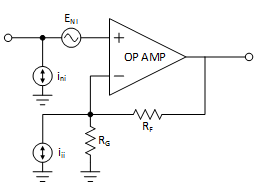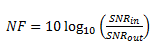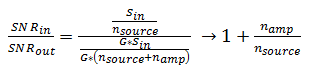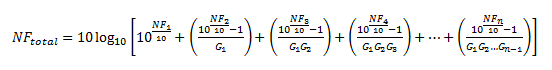# Disentangling RF amplifier specs: amplifier spot noise vs. noise figure

In the world of high-speed amplifiers, noise is commonly represented one of two ways. For radio frequency (RF)-oriented amplifiers, such as low-noise amplifiers (LNAs), radio frequency power amplifiers (PAs) and RF gain blocks, noise is usually given as a noise figure value. For high-speed operational amplifiers (op amps) and fully differential amplifiers (FDAs), noise is typically represented as voltage spot noise, which is sometimes also accompanied by current spot noise.

Both noise figure and spot noise are useful for their own reasons, but can be confusing if you are not familiar with one or the other. In order to reduce the mystery between the two specs, let’s take a look at how they are defined and related to one another.

Spot noise is the more straightforward of the two specs: It is simply a way to represent the noise of the amplifier as a source at one of the summing inputs. It is defined as the amplifier noise integrated over a one hertz bandwidth. The units are typically nanovolts per root hertz for voltage noise and amps per root hertz for current noise.

Voltage spot noise is represented by a voltage source that can be applied at either input of the amplifier, while current spot noise is represented by either one current source applied to each input or by specific sources for the inverting and noninverting inputs, respectively. Most amplifiers typically have only a voltage-noise value or one voltage- and one current-noise value. This is because the difference in current noises at the inputs of the amplifier is usually minimal; and in general, current noise is insignificant compared to voltage noise. Current noise only becomes a dominating factor in current feedback-style amplifiers, or when the feedback resistor (RF) becomes very large. Figure 1 shows an op-amp circuit with equivalent amplifier voltage and noise sources.Figure 1: Op amp showing equivalent input-voltage and noise sources

Since spot noise is an absolute measurement, you can use it to easily compare the noise contributions of different amplifiers independent of their configuration. However, not all amplifier data sheets include spot noise measurements. Sometimes noise measurements are represented only as a noise figure. Noise figure can be a very useful specification, but you must first understand how it is defined in order to use it properly.

The key aspect to understand about noise figure is that it is a measurement made relative to the source impedance of the system’s input signal. This is true for noise-figure measurements of any device, not just amplifiers. The noise figure is defined as the ratio of the signal-to-noise ratio (SNR) at the output of a device to the SNR at the input, expressed in decibels (Equation 1):(1)

Thinking about this equation from a conceptual standpoint, it becomes easy to see how the noise figure becomes dependent on the source impedance of the system. Expressing the SNR in simple terms of signal, noise and gain, Equation 2 shows that increasing the source noise (most easily done by increasing source impedance) reduces the noise figure:(2)

This conclusion is backed up by data when computing the noise figure for a specific amplifier. Figure 2 shows the noise-figure values for the LMH5401 FDA with four different source resistances. In Figure 2, it is very easy to see that noise figure is indeed reducing as source impedance is increasing, even though the amplifier voltage and current spot noises have not changed.Figure 2: LMH5401 example of noise figure dependency on source impedance

From this analysis, it’s clear to see that noise figure is much more confusing than spot noise measurements. So why would anyone use it in the first place? Employed correctly, noise figure can be a very useful tool in determining total system noise quickly. If a system comprises multiple blocks of identically matched source and load impedances, you can use the respective noise figures of each block to easily calculate the total noise figure of the system using the Friis formula for noise. Equation 3 shows the Friis formula in terms of noise figure (NF) and gain (G) of blocks 1 though n of a system.(3)

For amplifiers, both spot noise and noise figure are useful specifications if you need to understand the noise contribution to a system. However, it is important to keep in mind the differences between the two specs. In general, spot noise is the easiest to use when comparing noise performance because it is an absolute measurement. You can also use noise figure to compare noise performance, but remember that it is relative to the source impedance of the measurement. If the source impedances are the same, you can use noise figure to compare the noise performance of different amplifiers, while additionally providing an easy way to estimate the noise impact of a block in a system.